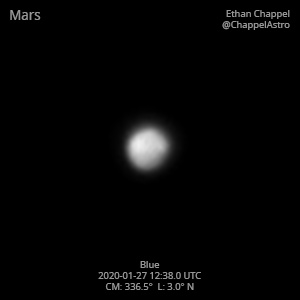# Mars 2020-01-27 12:38 UTC

CM1: 336.50°

CLat: +3.00°

Description

Started the day with the scariest event I've ever experienced: waking up to an alarm. I had the phone set to full volume and laying next to my ear. My heart was still racing at the end of the imaging session, making it slightly difficult to precisely focus the telescope.

Notes:

• Syrtis Major, which is setting, has a water-ice cloud over it.
• Sinus Sabeaus is at the central meridian.

Logs
```FireCapture v2.6  Settings
------------------------------------
Observer=Ethan Chappel
Camera=ZWO ASI174MM
Filter=B
Profile=Mars
Diameter=4.72"
Magnitude=1.40
CM=338.4°  (during mid of capture)
FocalLength=7700mm (F/27)
Resolution=0.16"
Filename=2020-01-27-1244_5-EC-B-Mars.ser
Date=2020_01_27
Start=12_43_02.310
Mid=12_44_32.313
End=12_46_02.316
Start(UT)=12_43_02.310
Mid(UT)=12_44_32.313
End(UT)=12_46_02.316
Duration=180.006s
Date_format=yyyy_MM_dd
Time_format=HH_mm_ss
LT=UT -6h
Frames captured=5137
File type=SER
Binning=no
Bit depth=8bit
Debayer=no
ROI=184x190
ROI(Offset)=0x0
FPS (avg.)=28
Shutter=35.00ms
Gain=400 (100%)
SoftwareGain=10 (off)
AutoExposure=off
AutoHisto=75 (off)
FPS=100 (off)
AutoGain=off
USBTraffic=80 (off)
Brightness=1 (off)
HighSpeed=off
Gamma=50 (off)
Histogramm(min)=0
Histogramm(max)=63
Histogramm=24%
Noise(avg.deviation)=2.46
AutoAlign=false
PreFilter=none
Limit=180 Seconds
Sensor temperature=23.3°C
FireCapture v2.6  Settings
------------------------------------
Observer=Ethan Chappel
Camera=ZWO ASI174MM
Filter=B
Profile=Mars
Diameter=4.72"
Magnitude=1.40
CM=335.2°  (during mid of capture)
FocalLength=8450mm (F/30)
Resolution=0.14"
Filename=2020-01-27-1231_4-EC-B-Mars.ser
Date=2020_01_27
Start=12_29_56.082
Mid=12_31_26.091
End=12_32_56.100
Start(UT)=12_29_56.082
Mid(UT)=12_31_26.091
End(UT)=12_32_56.100
Duration=180.018s
Date_format=yyyy_MM_dd
Time_format=HH_mm_ss
LT=UT -6h
Frames captured=5992
File type=SER
Binning=no
Bit depth=8bit
Debayer=no
ROI=184x190
ROI(Offset)=0x0
FPS (avg.)=33
Shutter=30.00ms
Gain=400 (100%)
SoftwareGain=10 (off)
AutoExposure=off
AutoHisto=75 (off)
FPS=100 (off)
AutoGain=off
USBTraffic=80 (off)
Brightness=1 (off)
HighSpeed=off
Gamma=50 (off)
Histogramm(min)=0
Histogramm(max)=86
Histogramm=33%
Noise(avg.deviation)=2.47
AutoAlign=false
PreFilter=none
Limit=180 Seconds
Sensor temperature=22.7°C
```